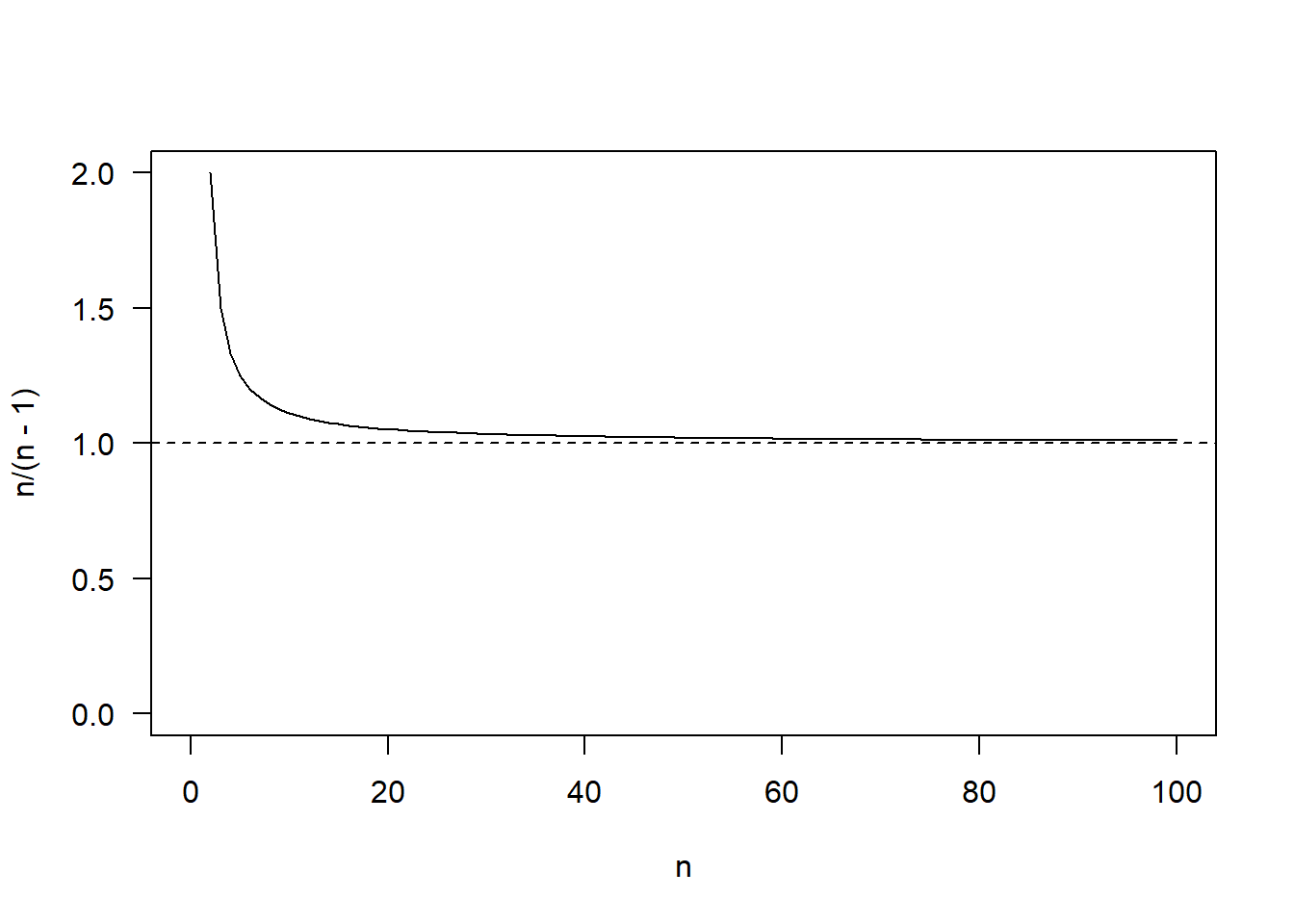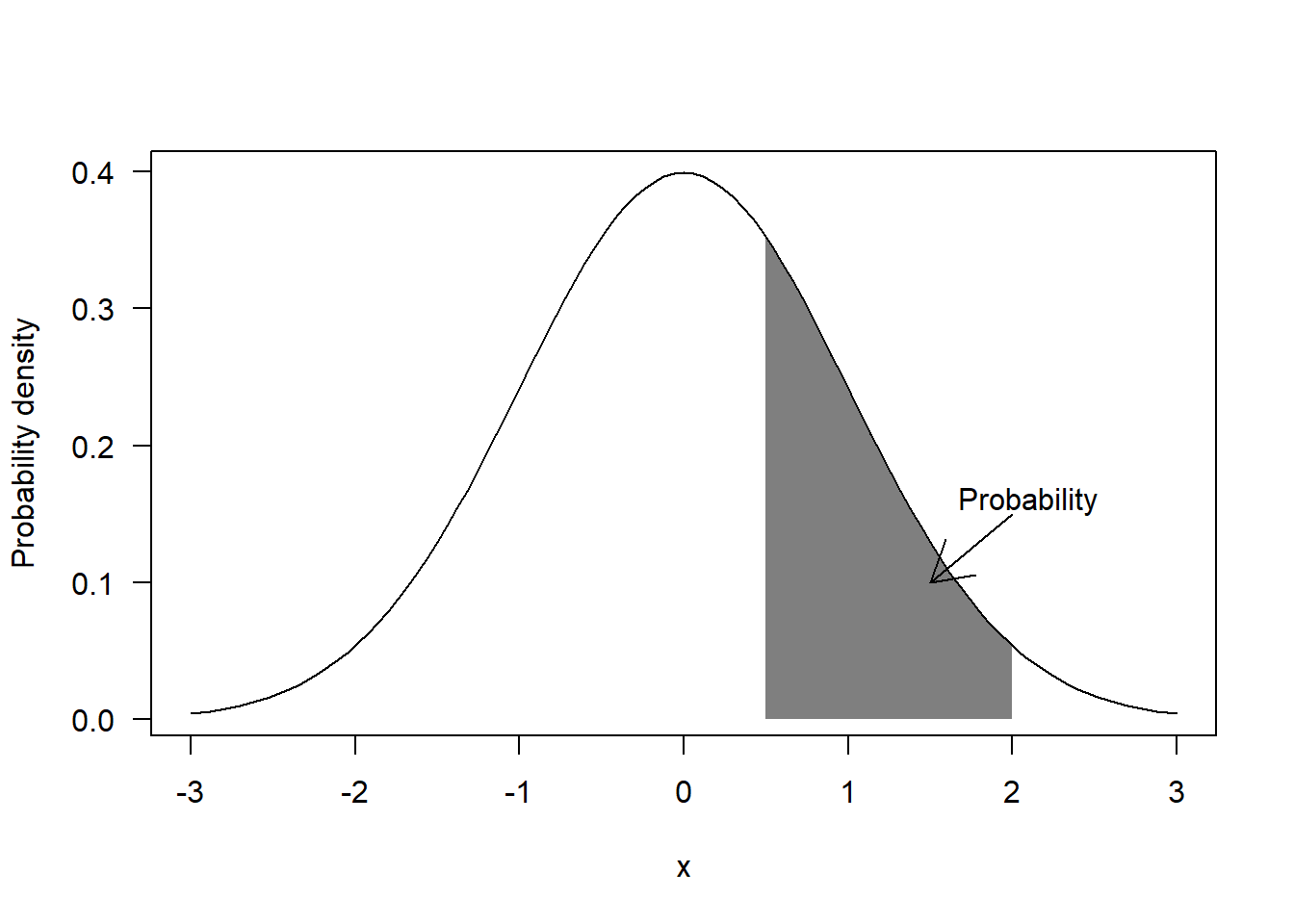# Articles by R on Alejandro Morales' Blog

### Estimating variance: should I use n or n – 1? The answer is not what you think

August 31, 2019 |

Estimates of population parameters based on samples are not exact: there is always some error involved. In principle, one can estimate a population parameter with any estimator, but some will be better than others. There is one particular case which was always very confusing to me (because of the multiple ...### Maximum likelihood estimation from scratch

August 25, 2019 |

Maximum likelihood estimates of a distribution Maximum likelihood estimation (MLE) is a method to estimate the parameters of a random population given a sample. I described what this population means and its relationship to the sample in a previous post. Before we can look into MLE, we first need to ...### Beyond normality: the bootstrap method for hypothesis testing

August 17, 2019 |

tl;dr: Parametric bootstrap methods can be used to test hypothesis and calculate p values while assuming any particular population distribution we may want. Non-parametric bootstrapping methods can be used to test hypotheses and calculate p values without having to assume any particular population as long as the sample can ... [Read more...]

### Where do p-values come from? Fundamental concepts and simulation approach

August 9, 2019 |

tl;dr: P-values are tail probabilities calculated from the sampling distribution of a sample-based statistic. This sampling distribution will depend on the size of the sample, the statistic being calculated and assumptions about the random population from which the data could have been sampled. For a few cases, analytical p-values ...